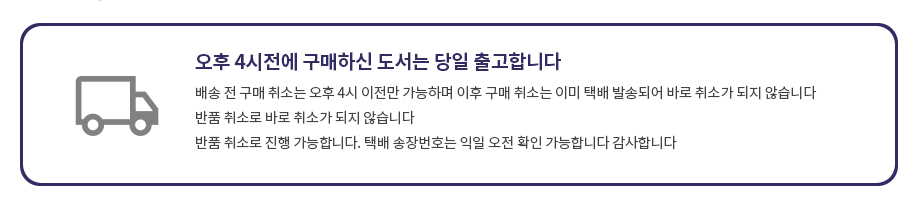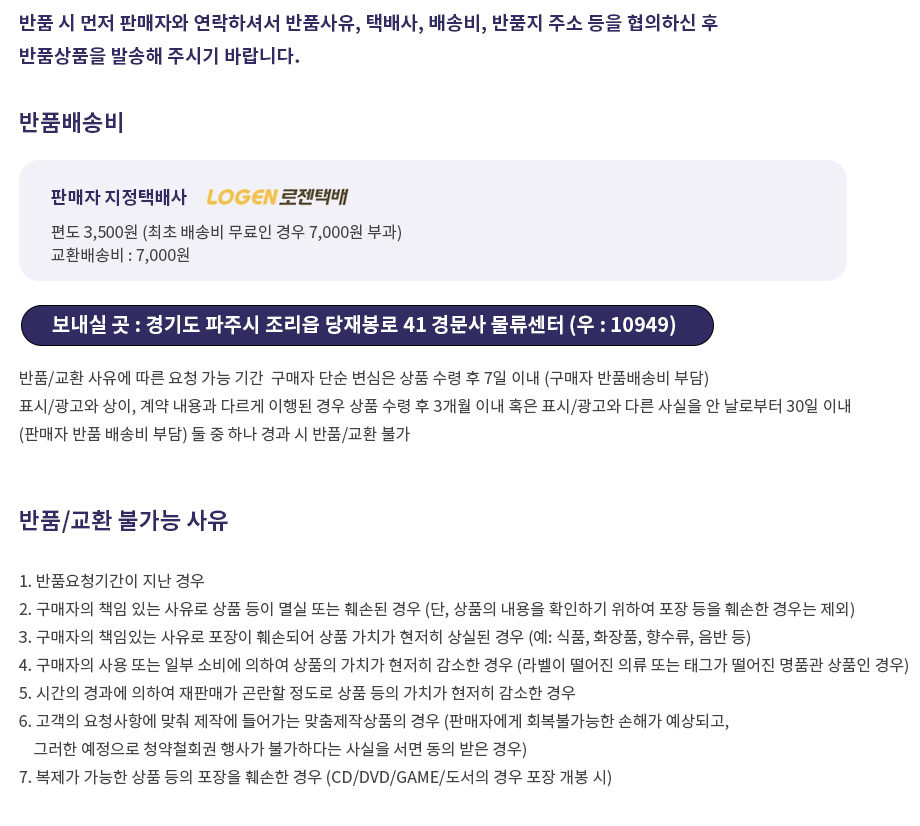## 로그인이필요합니다## 도서를 검색해 주세요.

원하시는 결과가 없으시면 문의 주시거나 다른 검색어를 입력해보세요.

•상품상세

## Probability: Modeling and Applications to Random Processes (2006) 요약정보 및 구매

상품 선택옵션 0 개, 추가옵션 0 개

사용후기 0 개
지은이 Miller 2006-08-09 1판 459 9780471458920 구매가능 15,000원 0점 주문시 결제

### 선택된 옵션

• Probability: Modeling and Applications to Random Processes (2006)
+0원

## 관련상품

• Improve Your Probability of Mastering This Topic This book takes an innovative approach to calculus-based probability theory, considering it within a framework for creating models of random phenomena. The author focuses on the synthesis of stochastic models concurrent with the development of distribution theory while also introducing the reader to basic statistical inference. In this way, the major stochastic processes are blended with coverage of probability laws, random variables, and distribution theory, equipping the reader to be a true problem solver and critical thinker. Deliberately conversational in tone, Probability is written for students in junior- or senior-level probability courses majoring in mathematics, statistics, computer science, or engineering. The book offers a lucid and mathematicallysound introduction to how probability is used to model random behavior in the natural world. The text contains the following chapters: * Modeling * Sets and Functions * Probability Laws I: Building on the Axioms * Probability Laws II: Results of Conditioning * Random Variables and Stochastic Processes * Discrete Random Variables and Applications in Stochastic Processes * Continuous Random Variables and Applications in Stochastic Processes * Covariance and Correlation Among Random Variables Included exercises cover a wealth of additional concepts, such as conditional independence, Simpson's paradox, acceptance sampling, geometric probability, simulation, exponential families of distributions, Jensen's inequality, and many non-standard probability distributions.
• Chapter 1. Modeling. 1.1 Choice and Chance. 1.2 The Model Building Process. 1.3 Modeling in the Mathematical Sciences. 1.4 A First Look at a Probability Model: The Random Walk. 1.5 Brief Applications of Random Walks. Exercises. Chapter 2. Sets and Functions. 2.1 Operations with Sets. 2.2 Functions. 2.3 The Probability Function and the Axioms of Probability. 2.4 Equally Likely Sample Spaces and Counting Rules. Rules. Exercises. Chapter 3. Probility Laws I: Building on the Axioms. 3.1 The Complement Rule. 3.2 The Addition Rule. 3.3 Extensions and Additional Results. Exercises. Chapter 4. Probility Laws II: Results of Conditioning. 4.1 Conditional Probability and the Multiplication Rule. 4.2 Independent Events. 4.3 The Theorem of Total Probabilities and Bayes' Rule. 4.4 Problems of Special Interest: Effortful Illustrations of the Probability Laws. Exercises. Chapter 5. Random Variables and Stochastic Processes. 5.1 Roles and Types of Random Variables. 5.2 Expectation. 5.3 Roles, Types, and Characteristics of Stochastic Processes. Exercises. Chapter 6. Discrete Random Variables and Applications in Stochastic Processes. 6.1 The Bernoulli and Binomial Models. 6.2 The Hypergeometric Model. 6.3 The Poisson Model. 6.4 The Geometric and Negative Binomial. Models. Exercises. Chapter 7. Continuous Random Variables and Applications in Stochastic Processes. 7.1 The Continuous Uniform Model. 7.2 The Exponential Model. 7.3 The Gamma Model. 7.4 The Normal Model. Chapter 8. Covariance and Correlation Among Random Variables. 8.1 Joint, Marginal and Conditional Distributions. 8.2 Covariance and Correlation. 8.3 Brief Examples and Illustrations in Stochastic Processes and Times Series. Exercises. Bibliography. Tables. Index.
• 학습자료

등록된 학습자료가 없습니다.

정오표

등록된 정오표가 없습니다.

사용후기가 없습니다.

상품문의가 없습니다.

• ## 배송/교환정보

### 배송정보### 교환/반품 정보### 선택된 옵션

• Probability: Modeling and Applications to Random Processes (2006)
+0원